# Year 11 Revision - Level 1

This is a level 1 revision exercise to help prepare for Year 11/end of Key Stage 4 tests.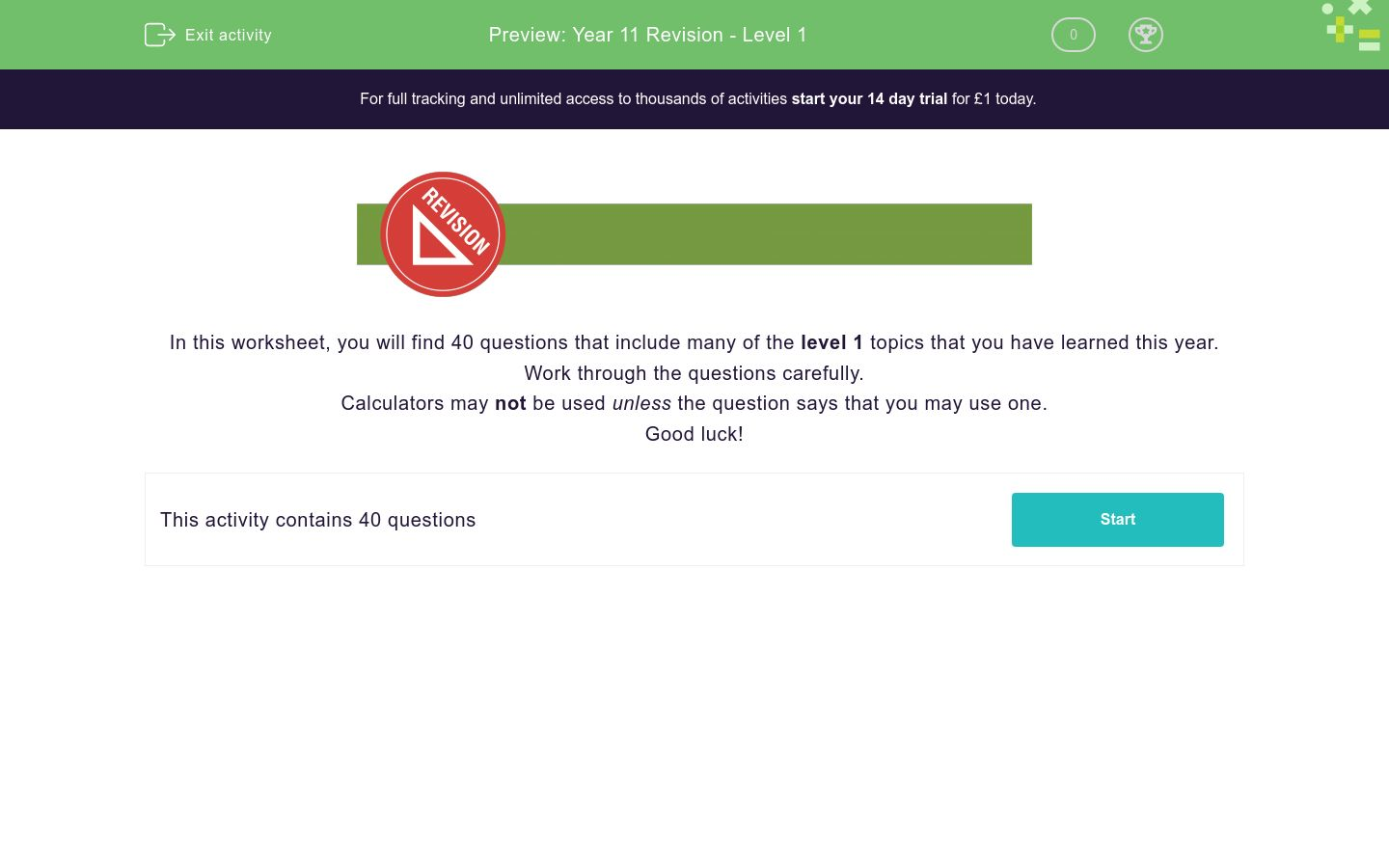Key stage:  KS 4

Curriculum topic:  Revision

Curriculum subtopic:  Year 11 Revision

Difficulty level:### QUESTION 1 of 10In this worksheet, you will find 40 questions that include many of the level 1 topics that you have learned this year.

Work through the questions carefully.

Calculators may not be used unless the question says that you may use one.

Good luck!

Work out:

3 - 0.32

Is the following number rational or irrational?

3

rational

irrationalYou may use a calculator for this question.

Select the recurring decimal which is equal to:

 4 331.

2.

3.

Work out, without a calculator:

√9 ×  33

Work out:

 7 - 5 + 7 15 6 10

(Give your answer as a fraction, reduced to its lowest terms, e.g. 7/10.)

Place these numbers in ascending order of size:

35%     1/3     3/10     √0.16     0.73

## Column B

1 (smallest)
35%
2
√0.16
3
0.73
4
3/10
5 (largest)
1/3

Round this number to 3 significant figures:

5212.5

4 × 106  +   6 × 104

1 × 1024

4.06 × 106

4.6 × 106

Rearrange the following equation so that it is in the form y = ...

2y - 4x = 8

y = 4 + 2x

y = 4 - 2x

y = 2x - 4

Rearrange the following formula to make h the subject.

A = ½bh

h = ½Ab

h = 2A/b

h = ½A/b

h = 2Ab

Solve the following equation for x:

2x + 3(x - 1) = 32

(Just write the number.)

x2 - 8x + 16

(x - 4) (x - 4)

(x - 2) (x - 8)

(x - 1) (x - 9)

(x + 16) (x - 1)

Is this an equation with one solution, an equation with no solutions or an identity?

3x + 12 = 3(x + 4)

equation with one solution

equation with no solutions

identity

Select the correct equation which is represented by graph B.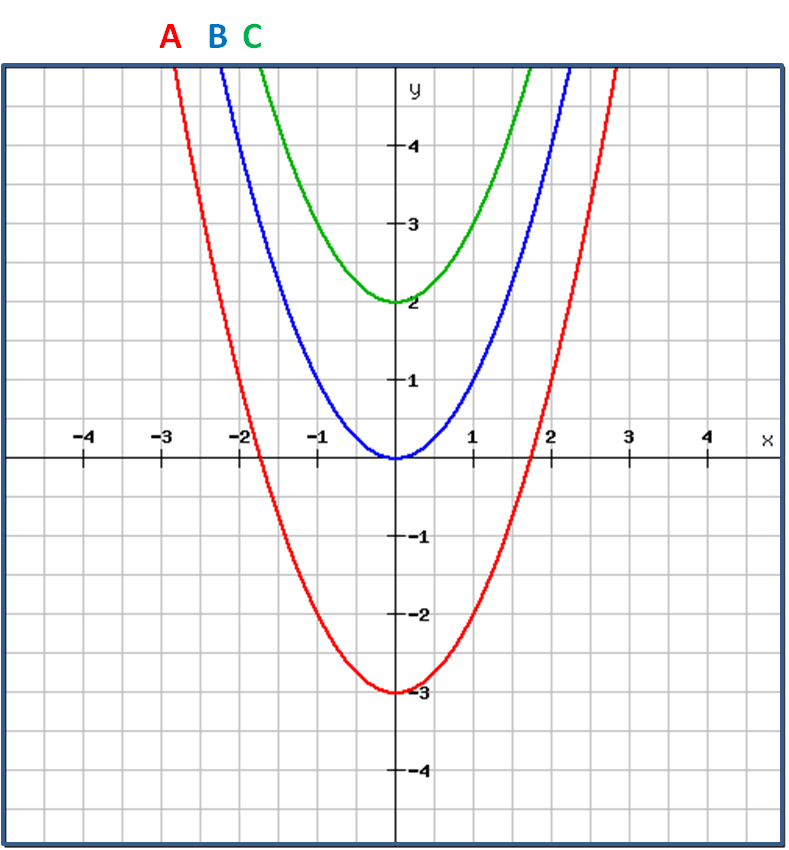y = x²

y = 2x²

y = x + 2

y = 2x

y = x³ + 2

Divide the following expression by  2.

4x4 - 6x3 + 10x2 - 2x

8x6 - 2x3 + 5x2 - x

2x4 - 3x3 + 5x2 - x

2x4 - 12x2 + 3x - 1

Choose the equation which represents a line with a gradient of 3 that passes through the point (3, -1).

3x + y = 10

y - 3x = -10

y + 3x = 10

y = -3x + 8

Select all the possible equations represented by the red line shown below.y = 4x - 5

x = 4y - 5

y + 5 = 4x

y = ¼x - 5

y = 5x - 5

Find the smallest integer that satisfies the inequality:

15 - 5x ≤ 2(1 - x)You may use a calculator for this question.

William invests £50 000 at 3% compound interest p.a.

He makes no further withdrawals or investments.

How much is his investment worth in pounds after five years?

(Just write the number with 2 decimal places.)

Given that y is directly proportional to x and that x = 8 when y = 192,

find the value of y when x = 25.

Given that y is inversely proportional to x and that x = 8 when y = 3,

find the value of y when x = 6.You may use a calculator for this question.

Look at the function:

f(t) = 3t + 10

Work out f(5).

Look at this graph.

Is it exponential, quadratic or linear?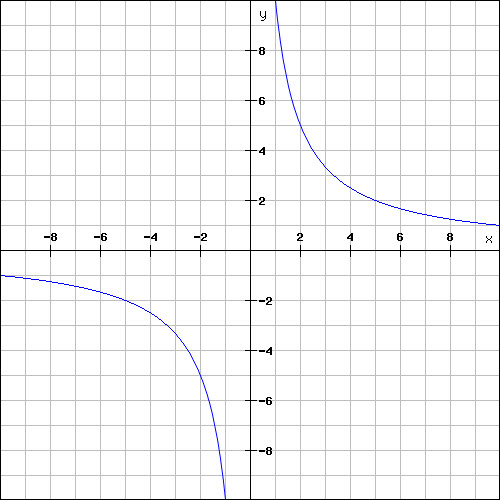exponential

linear

ABCDEFGH is a cuboid.

Select the coordinates of F.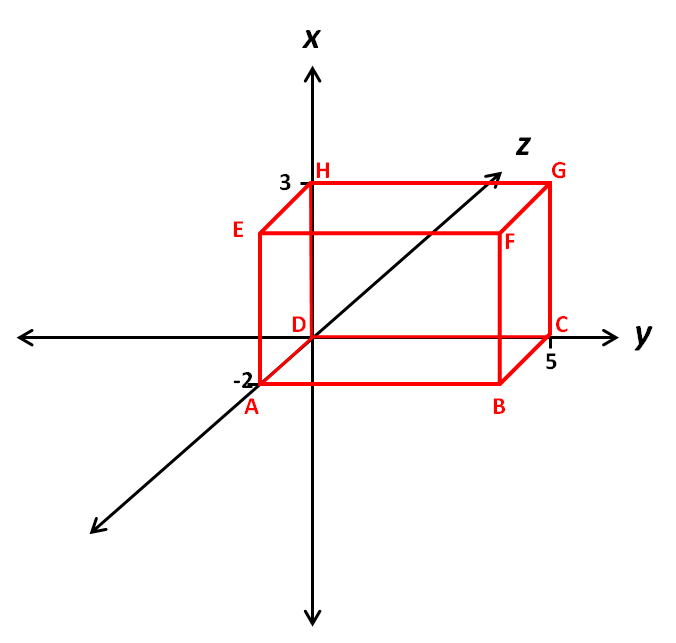(3, 5, 2)

(3, 5, -2)

(-2, 5, 3)You may use a calculator for this question.Calculate the volume (in cm3) of a cone with radius R equal to 2 cm and height H equal to 6 cm.

M and N are the midpoints of AB and BC respectively.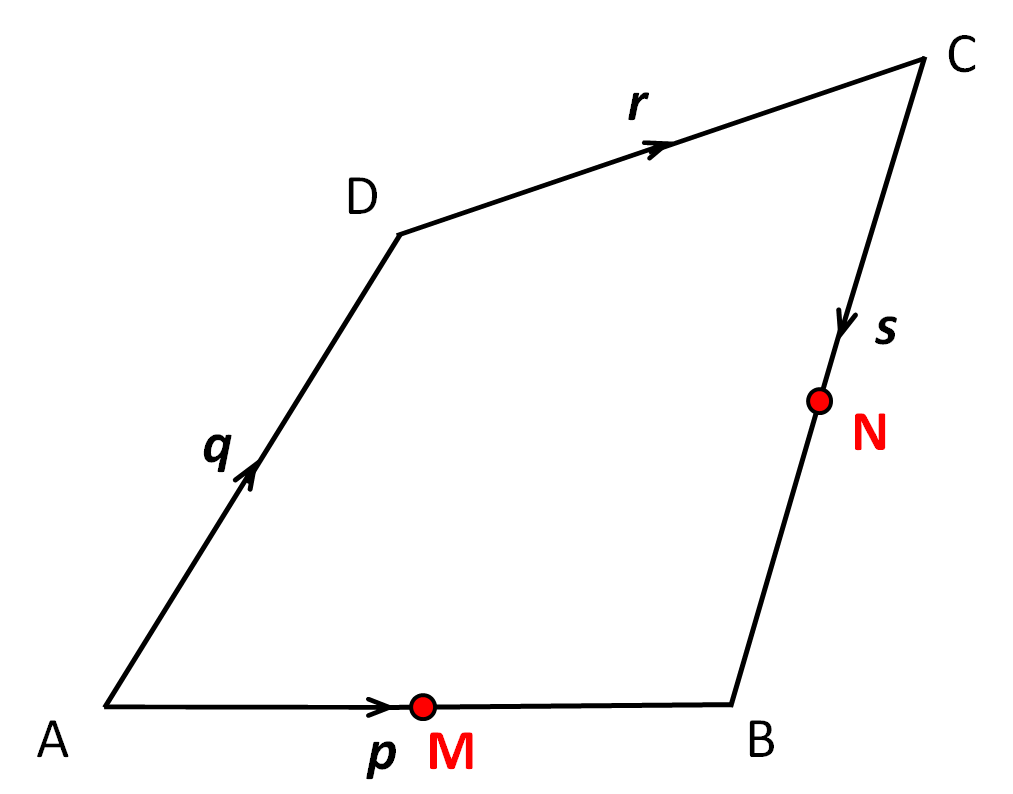Select an expression, in terms of p, q, r and s, for the vector½p - ½s

½p + ½s

-½p + s

½psYou may use a calculator for this question.

Calculate the perimeter of this right-angled triangle to 3 sig. figs.Using one of the triangles shown below, state the value of:

cos 45°2/√3

1

√2

1/√3

1/√2You may use a calculator for this question.

Using trigonometry, calculate the side length x to 3 sig. figs.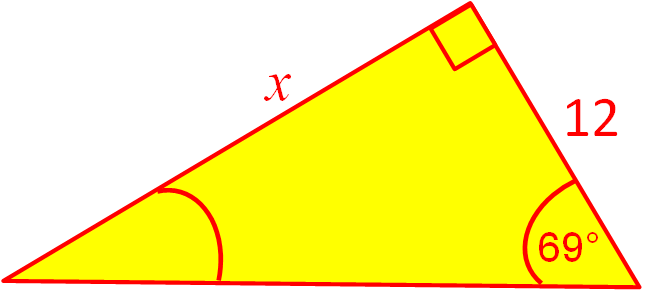You may use a calculator for this question.

Using trigonometry, calculate the angle θº to 3 sig. figs.

(Just write the number.)The tangent SU touches the circle, with centre C, at T.

Calculate the angle TUP in degrees.

(Just write the number.)ABC is a triangle, inside which is inscribed a circle.

The circle touches the triangle at the points P, Q and R.

AB = 23 and BQ =11.

Calculate the length AP.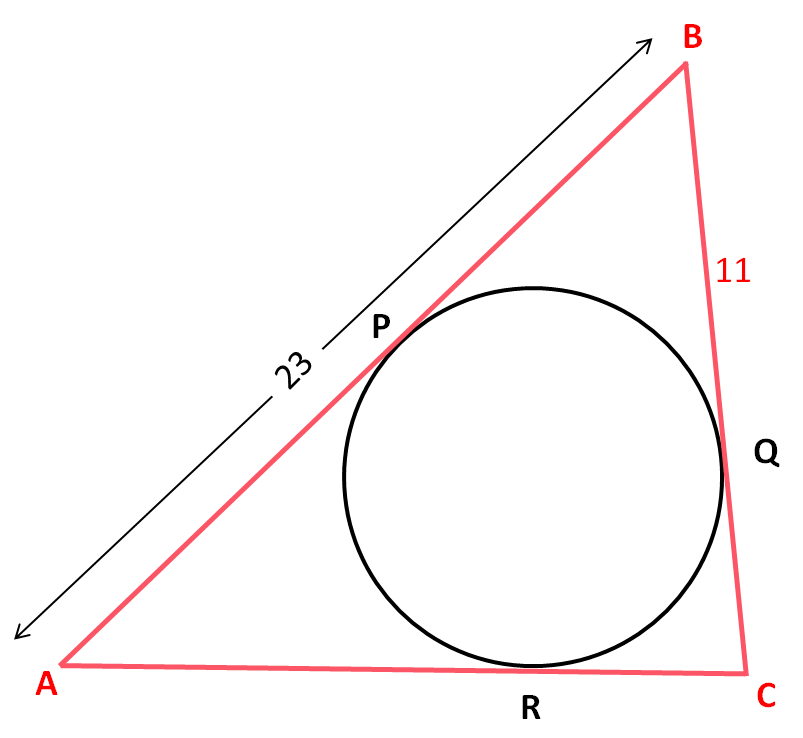A money bag contains 20 × £1 coins, 25 × 50p coins, 10 × 10p coins and 5 × 2p coins.

What is the probability that a coin selected at random is a 50p coin?

Two fair dice are thrown and the results are shown in this sample space diagram.What is the probability that the two dice will show the same numbers?

(Write your answer as a fraction in the form a/b in its lowest terms.)

A spinner was spun and the score was noted down before it was spun again.

The experiment was repeated several times.The results were as follows.

 Score Frequency 1 2 3 4 5 6 7 8 19 22 21 23 24 25 18 22

Calculate the expected probability of spinning a seven next.

(Give your answer as a fraction in its lowest terms in the form a/b.)

The probability that it will rain at the weekend is 6/24.

What is the probability that it will not rain at the weekend?

(Write your answer as a fraction in the form a/b in its lowest terms.)

Which of these statistical measures could be used to measure the spread of a set of data?

average

mean

median

mode

range

Look at these scatter graphs.

Match each situation to the most likely scatter graph.

ABC## Column B

Outside Temperature and No. of Ice Creams Sold
B
Shoe Size and Maths Test Marks
A
Distance Travelled and Petrol Left in the Tank
CYou may use a calculator for this question.

This chart shows the heights of children in a class at school.

Estimate the mean height of all the children in the class to the nearest cm.

(Just write the number.)The marks of 20 children in a maths test are represented on a Stem and Leaf Diagram as shown below.

Work out the median mark.

 Stem Leaf 0 7   7   9 1 1   2   4   4   4   4   7 2 1   3   3   3   9 3 0   4   5 4 4   8
• Question 1

Work out:

3 - 0.32

2.91
EDDIE SAYS
0.3 × 0.3 = 0.09
3 - 0.09 = 2.91
• Question 2

Is the following number rational or irrational?

3

irrational
EDDIE SAYS
It cannot be written as a fraction, so it is irrational.
• Question 3You may use a calculator for this question.

Select the recurring decimal which is equal to:

 4 331.
EDDIE SAYS
4 ÷ 33 = 0.1212121212...
The 1 and the 2 recur.
• Question 4

Work out, without a calculator:

√9 ×  33

81
EDDIE SAYS
√9 = 3
33 = 27
3 × 27 = 81
• Question 5

Work out:

 7 - 5 + 7 15 6 10

(Give your answer as a fraction, reduced to its lowest terms, e.g. 7/10.)

1/3
EDDIE SAYS
LCM of 15, 6 and 10 is 30.
14/30 - 25/30 + 21/30 = 10/30 = 1/3
• Question 6

Place these numbers in ascending order of size:

35%     1/3     3/10     √0.16     0.73

## Column B

1 (smallest)
3/10
2
1/3
3
0.73
4
35%
5 (largest)
√0.16
EDDIE SAYS
3/10 = 0.3
1/3 = 0.33333...
0.73 = 0.343
35% = 0.35
√0.16 = 0.4
• Question 7

Round this number to 3 significant figures:

5212.5

5210
EDDIE SAYS
5212.5 is between 5210 and 5220, but is closer to 5210.
• Question 8

4 × 106  +   6 × 104

4.06 × 106
EDDIE SAYS
4 × 106 = 4 000 000
6 × 104 = 60 000
4 000 000 + 60 000 = 4 060 000 = 4.06 × 106
• Question 9

Rearrange the following equation so that it is in the form y = ...

2y - 4x = 8

y = 4 + 2x
EDDIE SAYS
2y - 4x = 8
2y = 8 + 4x
y = 4 + 2x
• Question 10

Rearrange the following formula to make h the subject.

A = ½bh

h = 2A/b
EDDIE SAYS
Double both sides and then divide by b.
• Question 11

Solve the following equation for x:

2x + 3(x - 1) = 32

(Just write the number.)

7
EDDIE SAYS
2x + 3x - 3 = 32
5x = 35
x = 7
• Question 12

x2 - 8x + 16

(x - 4) (x - 4)
EDDIE SAYS
We look for factor pairs that multiply to give 16 and add to give -8.
These are -4 and -4.
Factorise x² - 4x - 4x + 16 in pairs.
• Question 13

Is this an equation with one solution, an equation with no solutions or an identity?

3x + 12 = 3(x + 4)

identity
EDDIE SAYS
3(x + 4) simplifies to 3x + 12 so this is true for all values of x.
• Question 14

Select the correct equation which is represented by graph B.y = x²
EDDIE SAYS
This is the basic y = x² parabolic curve.
• Question 15

Divide the following expression by  2.

4x4 - 6x3 + 10x2 - 2x

2x4 - 3x3 + 5x2 - x
EDDIE SAYS
 4x4 - 6x3 + 10x2 - 2x 2 2 2 2
• Question 16

Choose the equation which represents a line with a gradient of 3 that passes through the point (3, -1).

y - 3x = -10
EDDIE SAYS
y = mx + c is the equation and we know that the gradient m = 3.
Put x = 3 and y = -1 into y = 3x + c, to get -1 = 3 × 3 + c.
-1 = 9 + c gives c = -10.
Equation is y = 3x - 10 or y - 3x = -10.
• Question 17

Select all the possible equations represented by the red line shown below.y = 4x - 5
y + 5 = 4x
EDDIE SAYS
It crosses the y-axis at -5 and has a gradient of 4 (1 to the right and 4 up).
So y = mx + c becomes y = 4x - 5.
y + 5 = 4x is a rearrangement of this.
• Question 18

Find the smallest integer that satisfies the inequality:

15 - 5x ≤ 2(1 - x)

5
EDDIE SAYS
15 - 5x ≤ 2(1 - x)
15 - 5x ≤ 2 - 2x
15 - 2 ≤ 5x - 2x
13 ≤ 3x
3x ≥ 13
x ≥ 13/3 = 4.33333...
• Question 19You may use a calculator for this question.

William invests £50 000 at 3% compound interest p.a.

He makes no further withdrawals or investments.

How much is his investment worth in pounds after five years?

(Just write the number with 2 decimal places.)

57963.70
57 963.70
EDDIE SAYS
50 000 × 1.035
• Question 20

Given that y is directly proportional to x and that x = 8 when y = 192,

find the value of y when x = 25.

600
EDDIE SAYS
y = kx
192 = 8k
k = 192 ÷ 8 = 24
y = 24x
y = 24 × 25 = 600
• Question 21

Given that y is inversely proportional to x and that x = 8 when y = 3,

find the value of y when x = 6.

4
EDDIE SAYS
y = k/x
3 = k/8
k = 3 × 8 = 24
y = 24/x
y = 24 ÷ 6 = 4
• Question 22You may use a calculator for this question.

Look at the function:

f(t) = 3t + 10

Work out f(5).

253
EDDIE SAYS
35 = 243
243 + 10 = 253
• Question 23

Look at this graph.

Is it exponential, quadratic or linear?exponential
EDDIE SAYS
This is an exponential curve.
A linear graph would be straight.
• Question 24

ABCDEFGH is a cuboid.

Select the coordinates of F.(3, 5, -2)
EDDIE SAYS
3 along the x-axis.
5 along the y-axis.
-2 along the z-axis.
• Question 25You may use a calculator for this question.Calculate the volume (in cm3) of a cone with radius R equal to 2 cm and height H equal to 6 cm.

25.1
EDDIE SAYS
Vol = 1 ÷ 3 × π × 2² × 6
• Question 26

M and N are the midpoints of AB and BC respectively.Select an expression, in terms of p, q, r and s, for the vector½p - ½s
EDDIE SAYS
This is the same as M to B and then B to N, which is half of p and then half of -s.
• Question 27You may use a calculator for this question.

Calculate the perimeter of this right-angled triangle to 3 sig. figs.26.5
EDDIE SAYS
a² + 7² = 11²
a² + 49 = 121
a² = 121 - 49 = 72
a = √72 = 8.49
Perimeter = 8.49 + 7 + 11 = 26.49 = 26.5
• Question 28

Using one of the triangles shown below, state the value of:

cos 45°1/√2
EDDIE SAYS
In the yellow triangle, cos 45° = adj/hyp = 1/√2.
• Question 29You may use a calculator for this question.

Using trigonometry, calculate the side length x to 3 sig. figs.31.3
EDDIE SAYS
x = 12 × tan69°
• Question 30You may use a calculator for this question.

Using trigonometry, calculate the angle θº to 3 sig. figs.

(Just write the number.)41.8
EDDIE SAYS
θ = sin-1(6/9)
• Question 31

The tangent SU touches the circle, with centre C, at T.

Calculate the angle TUP in degrees.

(Just write the number.)48
EDDIE SAYS
The tangent SU and the diameter PT meet at right angles,

so ∠PTU = 90°
∠TUP = 180° - 90° - 42° = 48°

• Question 32

ABC is a triangle, inside which is inscribed a circle.

The circle touches the triangle at the points P, Q and R.

AB = 23 and BQ =11.

Calculate the length AP.12
EDDIE SAYS
BP = BQ = 11
AP = AB - BP = 23 - 11 = 12
• Question 33

A money bag contains 20 × £1 coins, 25 × 50p coins, 10 × 10p coins and 5 × 2p coins.

What is the probability that a coin selected at random is a 50p coin?

5/12
EDDIE SAYS
There are 60 coins in the bag.
P(50p coin) = 25/60 = 5/12
• Question 34

Two fair dice are thrown and the results are shown in this sample space diagram.What is the probability that the two dice will show the same numbers?

(Write your answer as a fraction in the form a/b in its lowest terms.)

1/6
EDDIE SAYS
There are 6 doubles out of 36.
6/36 reduces to 1/6.
• Question 35

A spinner was spun and the score was noted down before it was spun again.

The experiment was repeated several times.The results were as follows.

 Score Frequency 1 2 3 4 5 6 7 8 19 22 21 23 24 25 18 22

Calculate the expected probability of spinning a seven next.

(Give your answer as a fraction in its lowest terms in the form a/b.)

3/29
EDDIE SAYS
It is not stated that this is a fair spinner, so you must use the results.
The experiment is carried out 19 + 22 + 21 + 23 + + 24 + 25 + 18 + 22 = 174 times.
18/174 reduces to 3/29.
• Question 36

The probability that it will rain at the weekend is 6/24.

What is the probability that it will not rain at the weekend?

(Write your answer as a fraction in the form a/b in its lowest terms.)

3/4
EDDIE SAYS
1 - 6/24 = 1 - 1/4 = 3/4
• Question 37

Which of these statistical measures could be used to measure the spread of a set of data?

range
EDDIE SAYS
The range is the greatest value - least value, which is a measure of how spread out the data is.
• Question 38

Look at these scatter graphs.

Match each situation to the most likely scatter graph.

ABC## Column B

Outside Temperature and No. of Ic...
A
Shoe Size and Maths Test Marks
C
Distance Travelled and Petrol Lef...
B
EDDIE SAYS
A shows a positive correlation. The higher the temperature, the more ice creams are sold.
B shows a negative correlation. The further the distance, the less petrol there is remaining in the tank.
C shows no correlation. There is no connection between shoe size and marks gained in a test.
• Question 39You may use a calculator for this question.

This chart shows the heights of children in a class at school.

Estimate the mean height of all the children in the class to the nearest cm.

(Just write the number.)136
EDDIE SAYS
The total estimated height of all the children is (2 × 117.5) + (6 × 127.5) + (5 × 132.5) + (4 × 137.5) + (3 × 142.5) + (2 × 147.5) + (3 × 152.5) = 3392.5 cm.
There are 2 + 6 + 5 + 4 + 3 + 2 + 3 = 25 children in the class.
Mean height = 3392.5 ÷ 25 = 135.7 cm
• Question 40

The marks of 20 children in a maths test are represented on a Stem and Leaf Diagram as shown below.

Work out the median mark.

 Stem Leaf 0 7   7   9 1 1   2   4   4   4   4   7 2 1   3   3   3   9 3 0   4   5 4 4   8
19
EDDIE SAYS
With 20 people, we are looking for the 10th and 11th mark when placed in order. These are 17 and 21, the mean of which is 19.
---- OR ----

Sign up for a £1 trial so you can track and measure your child's progress on this activity.

### What is EdPlace?

We're your National Curriculum aligned online education content provider helping each child succeed in English, maths and science from year 1 to GCSE. With an EdPlace account you’ll be able to track and measure progress, helping each child achieve their best. We build confidence and attainment by personalising each child’s learning at a level that suits them.